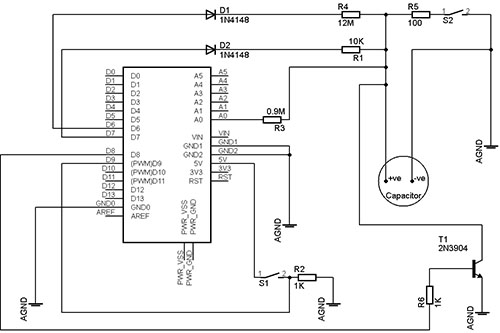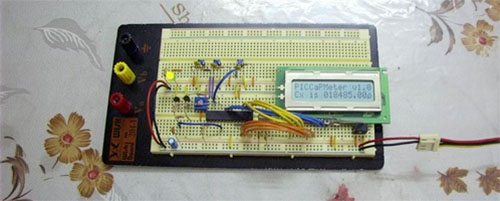Capacitor codes can be a nuisance to many getting acquainted with electronics – and capacitance measurement is still a somewhat rare feature among basic multimeters. Noting these troubles, Chris put an Arduino board to work –

Capacitors charge and discharge at certain ‘speeds’ based on the series resistance and its total capacitance. Skipping all of the semi-complicated maths, the time it takes for a capacitor to reach 63.2% of full charge is known as 1 RC time constant. The RC time constant is simply the product of the series resistance and the capacitance of the capacitor – R * C.
[…]
With this knowledge, I may be able to time how long it took for the capacitor to reach 63.2% charge, or, since they are proportional, 63.2% of the arduino’s voltage level. However, I don’t know how to implement a timer in the arduino language. I stuck with the 63.2% level but it didn’t have any physical (as in physics) relevance from then on. I had to rely on the fact that the value given by the Arduino will be proportional to the actual time constant (although no connection was made) and therefore the capacitance (fixed resistance). I knew that as the capacitance increased, so would the value output by the arduino.

Sounds like it could be quite handy for the 1ÂµF-2200ÂµF range – Capacitance Measurer v1.0

Related:Autorange capacitance meter

## 2 thoughts on “Arduino capacitance meter”

1.John R. Doner says:

I see that you’re charging the cap using a pin from the microprocessor as a voltage source. In order to use the RC time constant as an accurate guage of capacitance, the voltage source must be constant.

I don’t see anywhere in AtMega specs that the high output voltage of a pin, under varying load, will be constant.

Can you explain why this technique is accurate?